# 单智能体强化学习算法

## EC：Episodic Curiosity through Reachability

Posted by MY on August 5, 2020

## 二、解法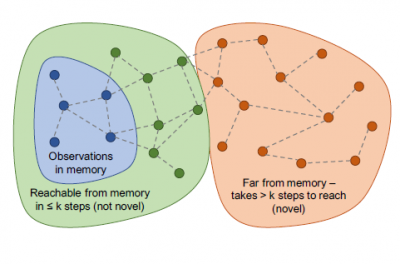### Episodic Curiosity算法框架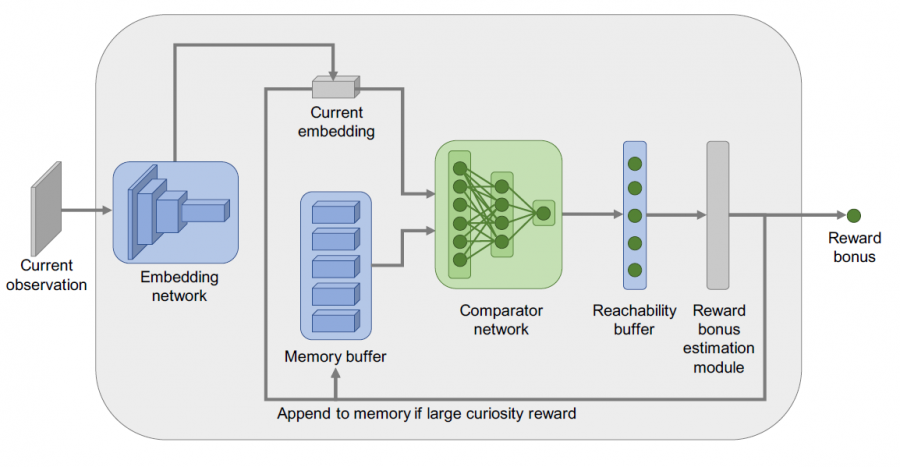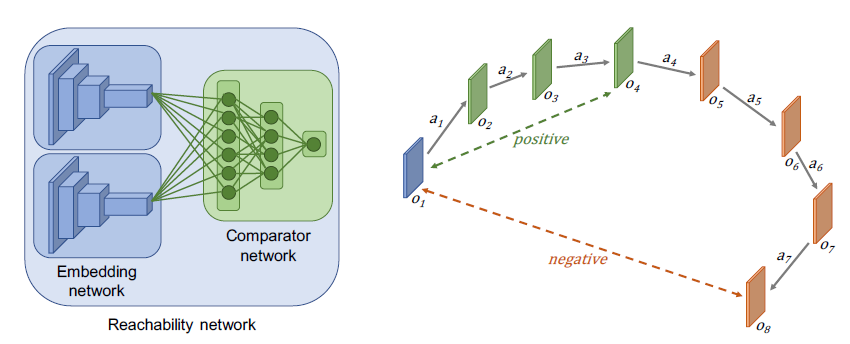$C(\mathbf{M}, \mathbf{e})=F\left(c_{1}, \ldots, c_{|\mathbf{M}|}\right) \in[0,1]$

$b=B(\mathbf{M}, \mathbf{e})=\alpha(\beta-C(\mathbf{M}, \mathbf{e}))$

### 网络训练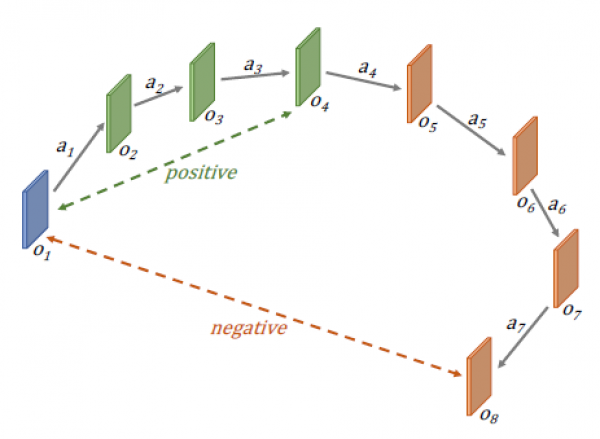## 三、实验内容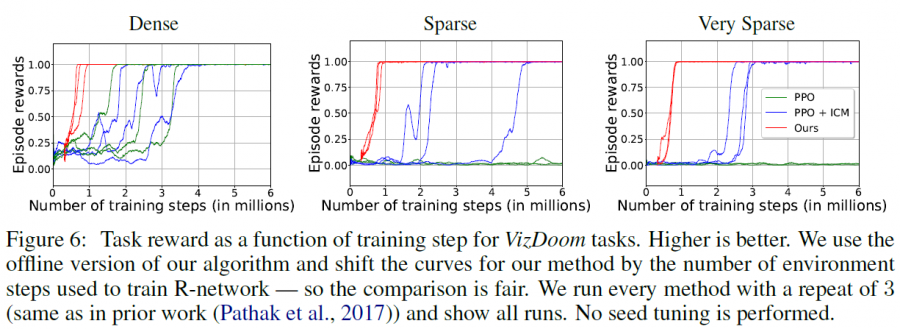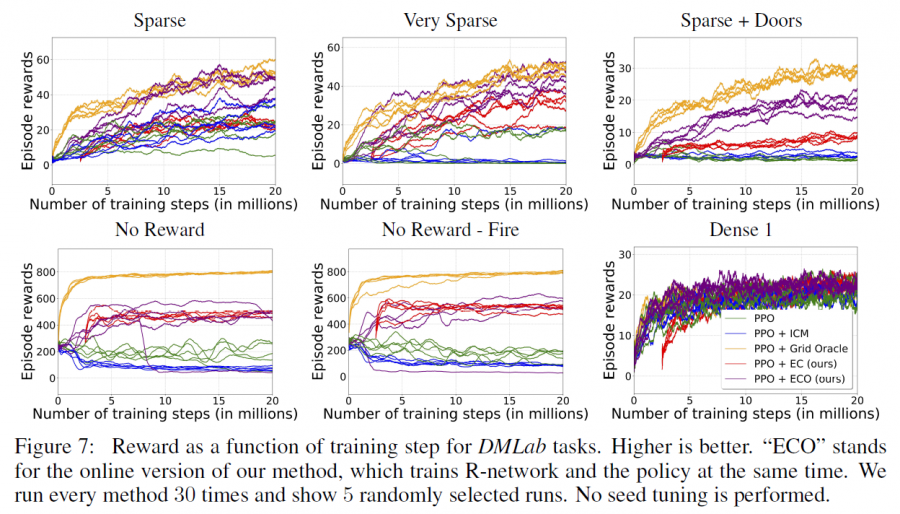## 五、优点

##### Share